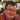# FCC: Sorted Union (JavaScript Intermediate Algorithm)

September 22, 2016

In FCC Challenge Sorted Union we’re tasked with flattening an array that has multiple arrays and removing duplicates in the array while still keeping the same array order.

``````function uniteUnique(arr) {
return arr
}

uniteUnique([1, 3, 2], [5, 2, 1, 4], [2, 1])``````

Because the number of arguments passed into the function is random we can’t use the arr variable. That variable only accesses the first array. We can access all the arrays passed in by using the arguments object. We can find out the number of arrays in the arguments object by using the built-in length function.

``````for (let x = 0; x < arguments.length; x += 1) {
array.push(arguments[x])
}``````

We then flatten the arrays by one level using the array’s built-in reduce function.

``````arr = array.reduce((a, b) => {
return a.concat(b)
})``````

We then use ECMAScript 6/2015’s new Set object to make the array elements unique without changing the order of the elements.

``````function uniq(a) {
return Array.from(new Set(a))
}``````

Rafase282 used JavaScript’s indexOf built-in function to determine whether an array element is unique. When indexOf returned -1 that means there’s no duplicate and then he pushes that array element into his own array.

``if (finalArray.indexOf(indexValue) < 0)``

My full code is below

``````function uniteUnique(arr) {
let array = []

for (let x = 0; x < arguments.length; x += 1) {
array.push(arguments[x])
}

arr = array.reduce((a, b) => {
return a.concat(b)
})

function uniq(a) {
return Array.from(new Set(a))
}

arr = uniq(arr)

console.log(arr)

return arr
}

uniteUnique([1, 3, 2], [5, 2, 1, 4], [2, 1])

uniteUnique([1, 3, 2], [1, ], [2, ])

uniteUnique([1, 2, 3], [5, 2, 1])

uniteUnique([1, 2, 3], [5, 2, 1, 4], [2, 1], [6, 7, 8])``````Written by Jia "Jay" Tan who lives and works in Las Vegas building useful things. You should follow him on Twitter